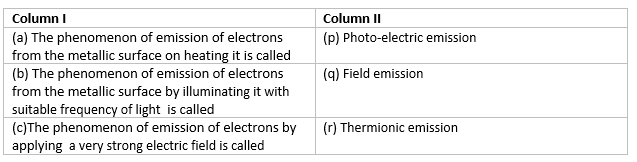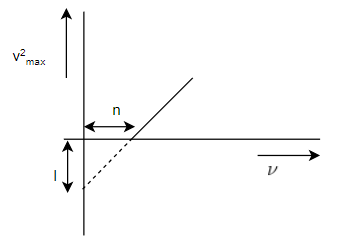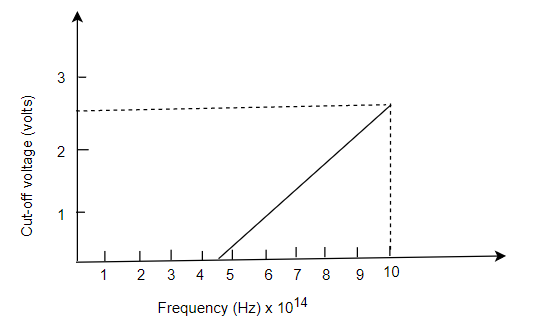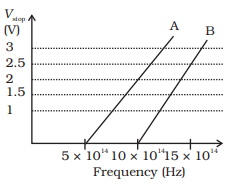# Dual Nature of Radiation and matter Questions

## Multiple Choice Questions

Question 1
An electron, an alpha-particle, and a proton have the same kinetic energy. Which of these particles has the shortest de Broglie wavelength?
(a) electron
(b) proton
(c) alpha-particle
(d) Cannot say

Question 2
How many electron volts make up 1 Joule
(a) $6.25 \times 10^{18}$ eV.
(b) $1.25 \times 10^{18}$ eV.
(c) $6.25 \times 10^{16}$ eV.
(d) $1.62 \times 10^{18}$ eV.

Question 3
What is the dimension of Work Function?
(a) $[ML^1T^{-2}]$
(b) $[ML^2T^{-3}]$
(c) $[ML^2T^{-1}]$
(d) $[ML^2T^{-2}]$

Question 4
if the maximum Kinetic energy of electrons emitted in a photo-cell is 6 eV.What is the stopping potential?
(a) 6 V
(b) 3 V
(c) 12 V
(d) 1 V

Question 5
A particle of a mass M at rest decays into two particles of masses $m_1$ and $m_2$ having non-zero velocities . What is the ratio of the de broglie wavelengths of the two particles $m_1$ and $m_2$
(A) 1 : 2
(B) 1 : 1
(C) 2 : 1
(D) 1 : 3

Question 6
The electrons ejected from metals due to effect of light are called
(a) primary electrons
(b) thermions
(c) secondary electrons
(d) photoelectrons

Question 7
The frequency of the incident light falling on a photosensitive metal plate is doubled,stopping potential will be
(a) Double the earlier value .
(b) Unchanged.
(c) Less than doubled.
(d) More than doubled.

Question 8
An electron is moving with an initial velocity $\mathbf{v}= v_0 \boldsymbol{i}$ and is in a magnetic field $\mathbf{B}= B_0 \boldsymbol{j}$. Then it�s de Broglie wavelength
(a) remains constant.
(b) increases with time.
(c) decreases with time.
(d) increases and decreases periodically

Question 9
The stopping potential ($V_0$) depends on
(a)the frequency of incident light
(b)the nature of the emitter material.
(c)For a given frequency of incident light, it is independent of its intensity
(d)All the above

Question 10
In photoelectric effect,the maximum velocity of the emitted electron depends on
(a) Intensity of the incident light
(b) Frequency of the incident light
(c) threshold frequency
(d) Both (b) and (c) above

Question 11
Photons absorbed in matter are converted to heat. A source emitting n photon/sec of frequency $\nu$ is used to convert 1kg of ice at 0°C to water at 0°C. Then, the time T taken for the conversion
(a) decreases with increasing n, with $\nu$ fixed.
(b) decreases with n fixed, $\nu$ increasing
(c) remains constant with n and $\nu$ changing such that $n \nu$ = constant.
(d) increases when the product $n \nu$ increases.

Question 12
A particle is dropped from a height H. The de Broglie wavelengthof the particle as a function of height is proportional to?
(a)$H^{-1/2}$
(b)$H$
(c)$H^{1/2}$
(d)$H^{2}$

Question 13
What is the de Broglie wavelength of an electron accelerated through a Potential difference of V volts
(a) $\lambda = \frac {1.227}{\sqrt {V}}$ nm
(b) $\lambda = 1.227 \sqrt {V}$ nm
(c) $\lambda = \frac {\sqrt {V}}{1.227}$ nm
(d) $\lambda = \frac {1.227}{V}$ nm

Question 14
Two metals A and B have work function 2eV and 4 eV respectively .which metal has a lower threshold wavelength for the photoelectric effect?
(a) A.
(b) B.
(c) Same for both.
(d) Cannot say.

Question 15(a) a -> p, b -> q , c -> r.
(b) a -> r, b -> q , c -> p.
(c) a -> r, b -> p , c -> q.
(d) a -> p, b -> r , c -> q.

## Text Based Questions(long)

Question 1
State the laws of photoelectric emission

Question 2
(i)Describe an experimental arrangement to study photoelectric effect?
(ii)Explain the effect of
(a)Intensity of light on photoelectric current
(b)Potential on photoelectric current
(c)Frequency of incident radiationon stopping potential

Question 3
(i)State Einstein's Photoelectric equation explaining the symobol used?
(ii) When a given photosensitive material is irradiated with light of frequency $\nu$, the maximum speed of the emitted photoelectrons equals $v^2_{max}$ . The square of $v_{max}$, i.e., $v^2_{max}$ , is observed to vary with $\nu$, as per the below Obtain expressions for (i) Planck's constant, and (ii) the work function of the given photosensitive material, in terms of the parameters l,n and the mass, m, of the electron.Question 4
What are de - Broglie waves? Write down de - Broglie wave equation.

Question 5
Explain giving reasons for the following.
(a) Photoelectric current in a photocell increases with the increase in the intensity of the incident radiation.
(b) The stopping potential $V_0$ varies linearly with the frequency $\nu$ of the incident radiation for a given photosensitive surface with the slope remaining the same for different surfaces.
(c) Maximum kinetic energy of the photo-electrons is independent of the intensity of incident radiation.

Question 1
Electrons are emitted from the cathode of a photocell of negligible work function, when photons of wavelength $\lambda$ are incident on it. derive the expression for the de broglie wavelength of the electrons emitted in terms of the wavelength of the incident light.

Question 2
Radiations of frequency $10^{15}$ Hz are incident on three photosensitive surfaces A , B and C
Following observations are recorded:
Surface A: No photoemission takes place.
Surface B: Photoemission takes place but photoelectrons have zero energy.
Surface C: Photoemission takes place but photoelectrons have some energy.
Explain the above observations on the basis of Einstein's photoelectric equation.?

Question 3
State how in a photo-cell, the work function of the metal influence the kinetic energy of the emitted electrons.
(a) If the intensity of the incident radiation is doubled, what change occur in (i) the stopping potential and (ii) in photoelectric current?
(b) If the frequency of the incident radiation is doubled, what changes occur in the (i) stopping potential and (ii) photoelectric current.

Question 4
An electron and proton have the same de broglie wavelength . Which one of these has the higher kinetic energy?. Which one is moving faster?

Question 5
What is mass of a photon of frequency $\nu$?

Question 6
A particle A with a mass $m_A$ is moving with a velocity v and hits a particle B (mass $m_B$) at rest (one dimensional motion). Find the change in the de Broglic wavelength of the particle A. Treat the collision as elastic

## Numerical Questions

Question 1
What is the de broglie wavelength associated with (i) an electron moving with a speed of $5.4 \times 10^6$ m/s and (ii) a ball of mass 1.50 g travelling at 30 m/s

Question 2
For photoelectric effect in sodium, fig. shows the plot of cut-off voltage versus frequency of the incident radiation.Calculate
i. Threshold frequency
ii. Workfunction

Question 3
A student performs an experiment on photoelectric effect, using two materials A and B. A plot of V stop vs ? is given in below figure(i) Which material A or B has a higher work function?
(ii) Given the electric charge of an electron = $1.6 \times 10^{-19}$ C, find the value of h obtained from the experiment for both A and B.
Comment on whether it is consistent with Einstein's theory:

Question 4
Monochromatic light of frequency $6.0 \times 10^{14}$ Hz is produced by a laser. The power emitted is $2.0 \times 10^{-3}$ W.
(a) What is the energy of a photon in the light beam?
(b) How many photons per second, on an average, are emitted by the source?

Question 5
When light of wavelength 400nm is incident on the cathode of photocell, the stopping potential recorded is 6V. If the wavelength of the incident light is to 600nm, calculate the new stopping potential.
Given $h=6.6 \times 10^{-34}$ Js,$c=3 \times 10^{8}$ m/s,$e=1.6 \times 10^{-19}$ C

Question 6
What is the Kinetic energy of the photo electrons ejected from a tungsten surface by UV light of wavelength 1940 Å ? Express the result in eV. The photoelectric threshold of tungsten is 2300 Å .
Ans. K=1 eV

Question 7
The work function of sodium is 2 eV.
(a) Find the maximum energy of the photo electrons ejected when a sodium surface is illuminated with light of 3105 Å.
(b) Calculate the maximum wavelength of the light that would produce photoelectric effect.
Ans. 2 eV , 6210 Å

Question 8
A small plate of metal (work function 1.17 eV) is placed at a distance 2 m from monochromatic light source of wavelength 4.8x 10-7 and power 1.0 watt. The light falls normally on plate. Find the number of photons striking the metal plate per square meter per second. If a constant uniform magnetic field of strength 10-4 Tesla is applied parallel to the metal surface , find the radius of the largest circular path followed by the emitted photo electrons.
Ans. 4.82 x1016 , 4.0 cm

Question 9
Calculate the wavelength of the wave associated with :
(a) 1 MeV photon
(b) 1 MeV proton
Ans. 1.242 x 10-10 cm, 2.84 x 10-12 cm

Question 10
The molecules of a certain gas at 320 K have a rms speed of .499 Km/sec. Calculate the De Brogli wavelength of these molecules. What is the gas?
Ans. 0.25Å , O16